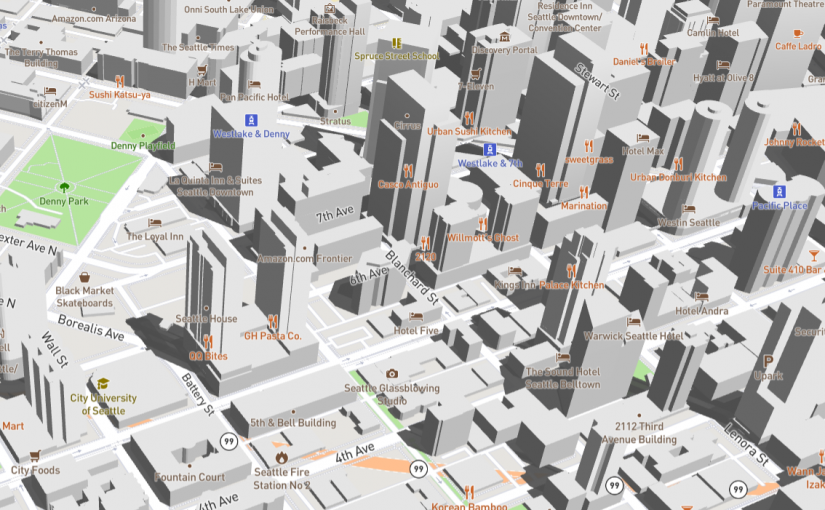# 建筑物阴影效果的实现

## 框架选择

### Cesium

```const viewer = new Cesium.Viewer("cesiumContainer", {
});

### Mapbox

```// vertexShader

uniform mat4 u_matrix;
uniform float u_height_factor;
uniform float u_altitude;
uniform float u_azimuth;
attribute vec2 a_pos;
attribute vec4 a_normal_ed;
attribute lowp vec2 a_base;
attribute lowp vec2 a_height;
void main() {
float base = max(0.0, a_base.x);
float height = max(0.0, a_height.x);
float t = mod(a_normal_ed.x, 2.0);
vec4 pos = vec4(a_pos, t > 0.0 ? height : base, 1);
float len = pos.z * u_height_factor / tan(u_altitude);
pos.x += cos(u_azimuth) * len;
pos.y += sin(u_azimuth) * len;
pos.z = 0.0;
gl_Position = u_matrix * pos;
}

void main() {
gl_FragColor = vec4(0.0, 0.0, 0.0, 0.7);
}```

vec4(a_pos, t > 0.0 ? height : base, 1)

pos.z * u_height_factor / tan(u_altitude)

pos.x += cos(u_azimuth) * len;
pos.y += sin(u_azimuth) * len;

## 发布者### Zhang

hope you enjoy my articles.